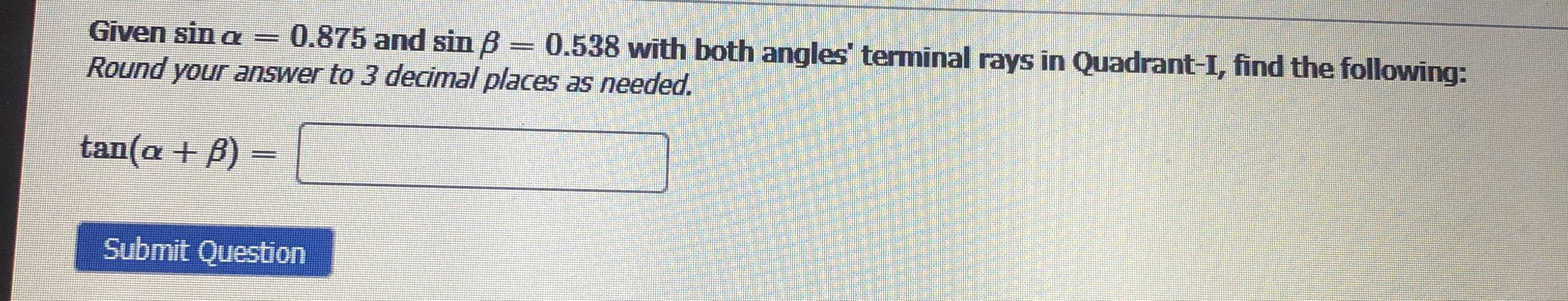### Still have math questions?

Trigonometry
QuestionGiven $$\sin \alpha = 0.875$$ and $$\sin \beta = 0.538$$ with both angles' terminal rays in Quadrant-I, find the following:

$$\tan ( \alpha + \beta ) =$$ _______

Round your answer to $$3$$ decimal places as needed.